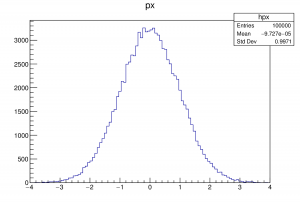# ROOT Histograms in Python

Apr, 16, 2017

ROOT is a data analysis framework developed by CERN that is well-suited for the analysis of certain large scientific data sets such as particle collision events and astronomical data.

One useful ROOT feature are the histograms. A simple program that histograms 100,000 Gaussian random numbers is shown here:

```
from __future__ import division, print_function
from ROOT import gRandom, TCanvas, TH1F

c1 = TCanvas('c1', 'Example', 200, 10, 700, 500)
hpx = TH1F('hpx', 'px', 100, -4, 4)

for i in xrange(100000):
px = gRandom.Gaus()
hpx.Fill(px)

hpx.Draw()
c1.Update()

# Save the plot as an image
c1.Print("myhistogram.eps")

```

It’s output is shown here:A second program is given on page~\pageref{root-histograms} that shows how the ROOT TCanvas can be split into regions to show multiple plots on the same canvas. It’s output is shown here:

We can also split ROOT’s TCanvas into regions to show multiple plots on a single canvas. A simple program that does that is shown here:

```
from __future__ import division, print_function
from ROOT import gRandom, TCanvas, TH1F

# Create a canvas
c1 = TCanvas('c1', 'My Basic Histograms', 200, 10, 700, 500)

# Divide the canvas into two plot areas
c1.Divide(1,2)

# Create a histograms
hist1 = TH1F('hist1', 'First', 100, -4, 4)
hist2 = TH1F('hist2', 'Second', 100, -4, 4)

for i in xrange(100000):
px = gRandom.Gaus()
hist1.Fill(px)

for i in xrange(100000):
px = gRandom.Gaus()
hist2.Fill(px)

# Go to plot area 1
c1.cd(1)

# Plot the first histogram
hist1.Draw()

# Go to plot area 2
c1.cd(2)

# Plot the second histogram
hist2.Draw()

c1.Update()

# Save the plot as an image
c1.Print("myhistogram.eps")

```
Posted in Python | Tagged as: ,

This site uses Akismet to reduce spam. Learn how your comment data is processed.

Follow